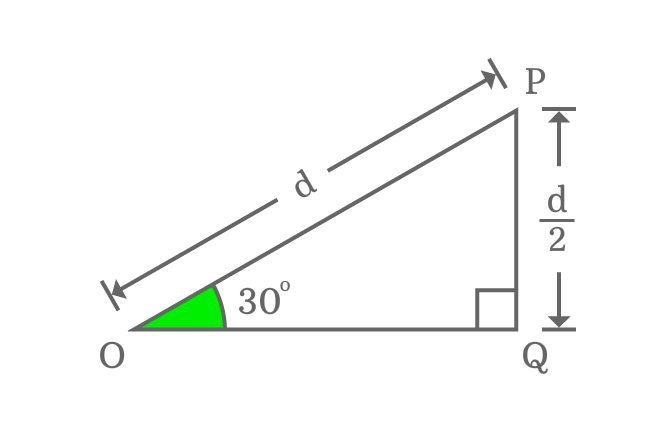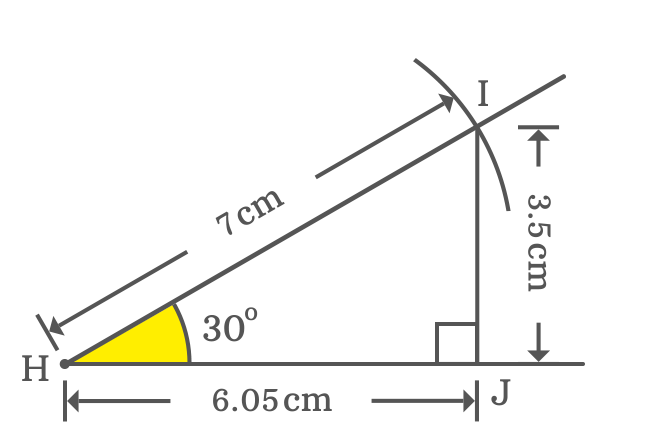# $\tan{(30^°)}$ Proof

The exact value of tan of 30 degrees in fraction form is $1/\sqrt{3}$. It can be derived mathematically in three approaches in which two of them are geometric approaches and third one is trigonometric approach. In trigonometry, the tan $30$ degrees value is derived on the basis of values of sin and cos of $30$ degrees.

### Theoretical approach

The exact value of $\tan{(30^°)}$ can be derived in trigonometry on the basis of geometrical relations between sides of the right triangle when the angle of the triangle is $\dfrac{\pi}{6}$. According to properties of right triangle, if the angle of right triangle is $30^°$, then the length of adjacent side is $\dfrac{\sqrt{3}}{2}$ times of length of hypotenuse.Therefore, $OQ = \dfrac{\sqrt{3}}{2} \times {OP}$ in the case of $\Delta POQ$

Now, express relation between all three sides of the triangle in mathematical form by Pythagorean Theorem.

${OP}^2 = {PQ}^2+{OQ}^2$

If $OQ = \dfrac{\sqrt{3}}{2} \times {OP}$ then $OP = \dfrac{2}{\sqrt{3}} \times {OQ}$. Now, substitute the length of hypotenuse by its equivalent value in the above equation.

$\implies$ ${\Bigg(\dfrac{2}{\sqrt{3}} \times {OQ}\Bigg)}^2 = {PQ}^2+{OQ}^2$

$\implies$ $\dfrac{4}{3}{OQ}^2 = {PQ}^2+{OQ}^2$

$\implies$ $\dfrac{4}{3}{OQ}^2-{OQ}^2 = {PQ}^2$

$\implies$ ${\Bigg(\dfrac{4}{3}-1\Bigg)}{OQ}^2 = {PQ}^2$

$\implies$ ${\Bigg(\dfrac{4-3 \times 1}{3}\Bigg)}{OQ}^2 = {PQ}^2$

$\implies$ ${\Bigg(\dfrac{4-3}{3}\Bigg)}{OQ}^2 = {PQ}^2$

$\implies$ ${\Bigg(\dfrac{1}{3}\Bigg)}{OQ}^2 = {PQ}^2$

$\implies$ $\dfrac{1}{3} = \dfrac{{PQ}^2}{{OQ}^2}$

$\implies$ $\dfrac{1}{3} = {\Bigg(\dfrac{PQ}{OQ}\Bigg)}^2$

$\implies$ ${\Bigg(\dfrac{PQ}{OQ}\Bigg)}^2 = \dfrac{1}{3}$

$\implies$ $\dfrac{PQ}{OQ} = \sqrt{\dfrac{1}{3}}$

$\implies$ $\dfrac{PQ}{OQ} = \dfrac{1}{\sqrt{3}}$

$PQ$ and $OQ$ are lengths of opposite and adjacent sides of the right triangle.

$\implies$ $\dfrac{Length \, of \, Opposite \, side}{Length \, of \, Adjacent \, side}$ $=$ $\dfrac{1}{\sqrt{3}}$

The angle of $\Delta POQ$ is $30$ degrees. So, the ratio represents tan of angle $30$ degrees according to definition of tan function.

$\,\,\, \therefore \,\,\,\,\,\, \tan{(30^°)} \,=\, \dfrac{1}{\sqrt{3}}$

$\implies \tan{(30^°)}$ $\,=\,$ $0.5773502691\ldots$

The exact value of tan of $30$ degrees is equal to $\dfrac{1}{\sqrt{3}}$ in fraction form and its value in decimal is $0.5773502691\ldots$

### Practical approach

You can even derive the value of tan of angle $30$ degrees by constructing a right triangle with $\dfrac{\pi}{6}$, using geometric tools but it is not possible to obtain the exact value of $\tan{{\Big(33\dfrac{1}{3}}^g\Big)}$ due to measuring lengths of sides approximately. However, its value approximately equals to the actual value.1. Consider a point in a plane and name it as point $H$. Draw a horizontal line from point $H$.
2. After that, take protractor and coincide its centre with point $H$ and also coincide its right side base line with horizontal line, drawn from point $H$. Now, identify $30^°$ and then mark it.
3. Take ruler and draw a straight line from point $H$ through point of $30^°$ angle.
4. Take the compass and set it to any length by ruler. In this case, $7 \, cm$. Later, draw an arc on $30^°$ angle line from point $H$ and it cuts the $30^°$ line at point $I$.
5. Draw a perpendicular line to horizontal line from point $I$ by Set Square to complete the construction of the right angled triangle with $30$ degrees.

In this way, the right triangle ($\Delta JHI$) with $\dfrac{\pi}{6}$ radians is constructed geometrically. Now, let’s find the value of $\tan{\Big(\dfrac{\pi}{6}\Big)}$ experimentally.

$\tan{(30^°)} = \dfrac{Length \, of \, Opposite \, side}{Length \, of \, Adjacent \, side}$

$\implies \tan{(30^°)} \,=\, \dfrac{IJ}{HJ}$Actually, the lengths of opposite side ($IJ$) and adjacent side ($HJ$) are unknown but they can be measured by ruler.

You will be observed that the length of opposite side is $3.5 \, cm$ exactly and the length of adjacent side lies between $6$ to $6.1 \, cm$. So, its length is considered as $6.05 \, cm$ approximately.

$\implies \tan{(30^°)} \,=\, \dfrac{IJ}{HJ} = \dfrac{3.5}{6.05}$

$\implies \tan{(30^°)} \,=\, \require{cancel} \dfrac{\cancel{3.5}}{\cancel{6.05}}$

$\,\,\, \therefore \,\,\,\,\,\, \tan{(30^°)} \,=\, 0.5785123967\ldots$

### Trigonometric approach

The exact value of $\tan{(30^°)}$ can also be evaluated in trigonometry by the quotient or ratio identity of sin and cos functions. In this case, the $\tan{\Big(\dfrac{\pi}{6}\Big)}$ value is actually calculated by the values of sin 30 degrees and cos 30 degrees.

$\tan{(30^°)} \,=\, \dfrac{\sin{(30^°)}}{\cos{(30^°)}}$

$\implies \tan{(30^°)} \,=\, \dfrac{\dfrac{1}{2}}{\dfrac{\sqrt{3}}{2}}$

$\implies \tan{(30^°)} \,=\, \dfrac{1}{2} \times \dfrac{2}{\sqrt{3}}$

$\implies \tan{(30^°)} \,=\, \dfrac{1 \times 2}{2 \times \sqrt{3}}$

$\implies \tan{(30^°)} \,=\, \dfrac{2}{2\sqrt{3}}$

$\implies \tan{(30^°)} \,=\, \require{cancel} \dfrac{\cancel{2}}{\cancel{2}\sqrt{3}}$

$\,\,\, \therefore \,\,\,\,\,\, \tan{(30^°)} \,=\, \dfrac{1}{\sqrt{3}}$

#### Verdict

The value of tan of $30$ degrees is $\dfrac{1}{\sqrt{3}}$ or $0.5773502691\ldots$ and is same according to both theoretical geometric and trigonometric methods but its value is $0.5785123967\ldots$ as per practical geometrical approach. They both are approximately equal but the value of $\tan{\Big(\dfrac{\pi}{6}\Big)}$ obtained from practical geometrical approach slightly differs with the other methods. It is due to measuring lengths of the sides with parallax error. It can’t be considered as actual value because it changes if you construct a right triangle practically with different lengths of the sides.

Latest Math Topics
Jun 26, 2023
Jun 23, 2023

Latest Math Problems
Jul 01, 2023
Jun 25, 2023
###### Math Questions

The math problems with solutions to learn how to solve a problem.

Learn solutions

Practice now

###### Math Videos

The math videos tutorials with visual graphics to learn every concept.

Watch now

###### Subscribe us

Get the latest math updates from the Math Doubts by subscribing us.